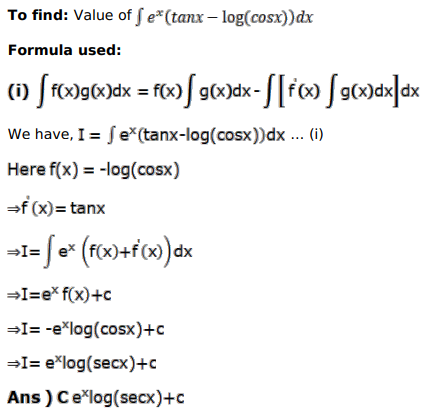# Mark against the correct answer in each of the following:

Question:

Mark $(\sqrt{ })$ against the correct answer in each of the following:

$\int e^{x}(\tan x-\log \cos x) d x=?$

A. $e^{x} \tan x+C$

B. $e^{x} \log \cos x+C$

C. $e^{x} \log \sec x+C$

D. none of these

Solution: